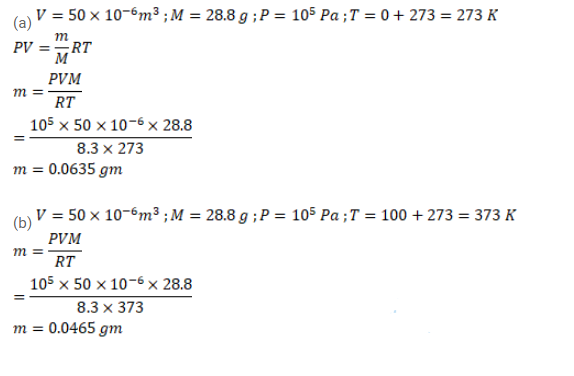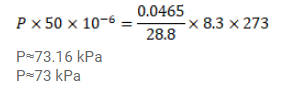# Use R=8.3 J/mol-K wherever required. A container of volume 50°C contains air (mean molecular weight=28.8 g)Question:

Use $\mathrm{R}=8.3 \mathrm{~J} / \mathrm{mol}-\mathrm{K}$ wherever required.

A container of volume $50^{\circ} \mathrm{C}$ contains air (mean molecular weight= $28.8 \mathrm{~g}$ ) and is open to atmosphere when the pressure is $100 \mathrm{kPa}$. The container is kept in a bath containing melting ice ( $\left.0^{\circ} \mathrm{C}\right)$.

(a) Find the mass of the air in the container when thermal equilibrium is reached.

(b) The container is now placed in another bath containing boiling water $\left(100^{\circ} \mathrm{C}\right.$ ). Find the mass of air in the container.

(c) The container is now closed and placed in the melting-ice bath. Find the pressure of the air when thermal equilibrium is reached.

Solution: